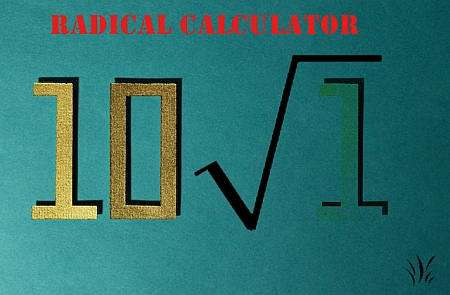Instructions: Use radical calculator to compute and simplify any expression involving radicals that you provide, showing all the steps. Please type in the radical expression you want to work out in the form box below.Enter the radical expression you want to compute (Ex: sqrt(2/3 + 4/5), etc.)

This calculator is a radical calculator with steps that will allow you to calculate and simplify a given radical expression you provide, showing all the steps of the process, or if the expression is already simplified, the calculator will tell you so.

So then, once the required expression has been typed in, all you need to do is click on the "Calculate" button to get all the relevant steps shown to you.

Radical expressions are very common in Algebra, especially when using square root. Other radical expressions are usually expressed in terms of the calculation of exponents, because of ease of notation.## How to simplify radical expressions?

Radical expressions are more than just dealing with square roots. There is usually some familiarity with operations involving square roots, like:

$\sqrt{x^4}$

for example. Most people would not have a problem with coming up with the right answer, which would be

$\sqrt{x^4} = x^2$

With radicals though, it is a little harder to interpret, for example, when you need to simplify something like $$\sqrt{x^4}$$. Usually the best strategy would be to express radicals in their equivalent power expression, where the exponents would do the job.

Specifically, we know that by definition: $$\sqrt[n]{a} = a^{1/n}$$, which gives a DIRECT link between radicals and powers.

## Steps for dealing with a radical form

• Step 1: Clearly identify the expression you want to calculate or simplify
• Step 2: Identify the type of root you have. Is it a basic square root, or is it another radical?
• Step 3: If you have a square root, chances are that simplification could be relatively easy, for which you can use this calculator
• Step 4: For other types of radicals, you want to use the fact that $$\sqrt[n]{a} = a^{1/n}$$ holds, and perhaps an appropriate strategy consists of expressing all the radicals as powers, and use the rule of exponents to simplify the expression

In practice, dealing with radical forms can be cumbersome, and even confusing, reason which converting all radicals to exponents and use the rules of exponents may be the best way to go.Using this calculator is simple, all you need to do is to correctly specify an expression that involves a radical, it could be a square root like 'sqrt(4x^2)', or it could be a more complex radical like 'sqrt(128x^8)'

You need to just type an expression that system recognizes as valid. Often times you can type directly the radical form in terms of exponents, like '(81 x^4)^(1/3)'.

## Why you should use a radical simplifier?

Because radical expressions appear very frequently in all realms of Algebra and Calculus. Usually, when solving equations you run into the need to dealing with radical forms to find a simpler form of an equation solution.

There are often times where you will need help with dividing radicals and showing steps, for which case the use of a calculator like this could prove invaluable. Mostly it is about time saving and double checking your own answers.### Example: Calculating the sum of fractions

Simplify the following radical form: $$\sqrt{81x^4 y^7}$$

Solution: In this case, it would be useful to express the radical term provided using exponents instead of radicals:

$\sqrt{81x^4 y^7} = \left(81x^4 y^7\right)^{1/3}$

Now, we rewrite the internal terms and simplify:

$\sqrt{81x^4 y^7} = \left(81x^4 y^7\right)^{1/3}$ $= \left(3^3 \cdot 3 x^3 \cdot x \cdot y^6 \cdot y\right)^{1/3}$ $= 3xy^2\left( 3x y\right)^{1/3}$ $= 3xy^2 \sqrt{3xy}$

which concludes the calculation.

## Other useful Algebra calculators

This calculator will be useful to you whenever you need to simplify a radical form. But there are lots of other types of expressions that you could potentially encounter.

If you need help with simplifying a fraction or doing a general simplification of an expression, calculators that show steps can make the whole difference..

There are different and multiple connections between different types of math objects, links between decimals and radicals, decimals and fractions, a too many to count.